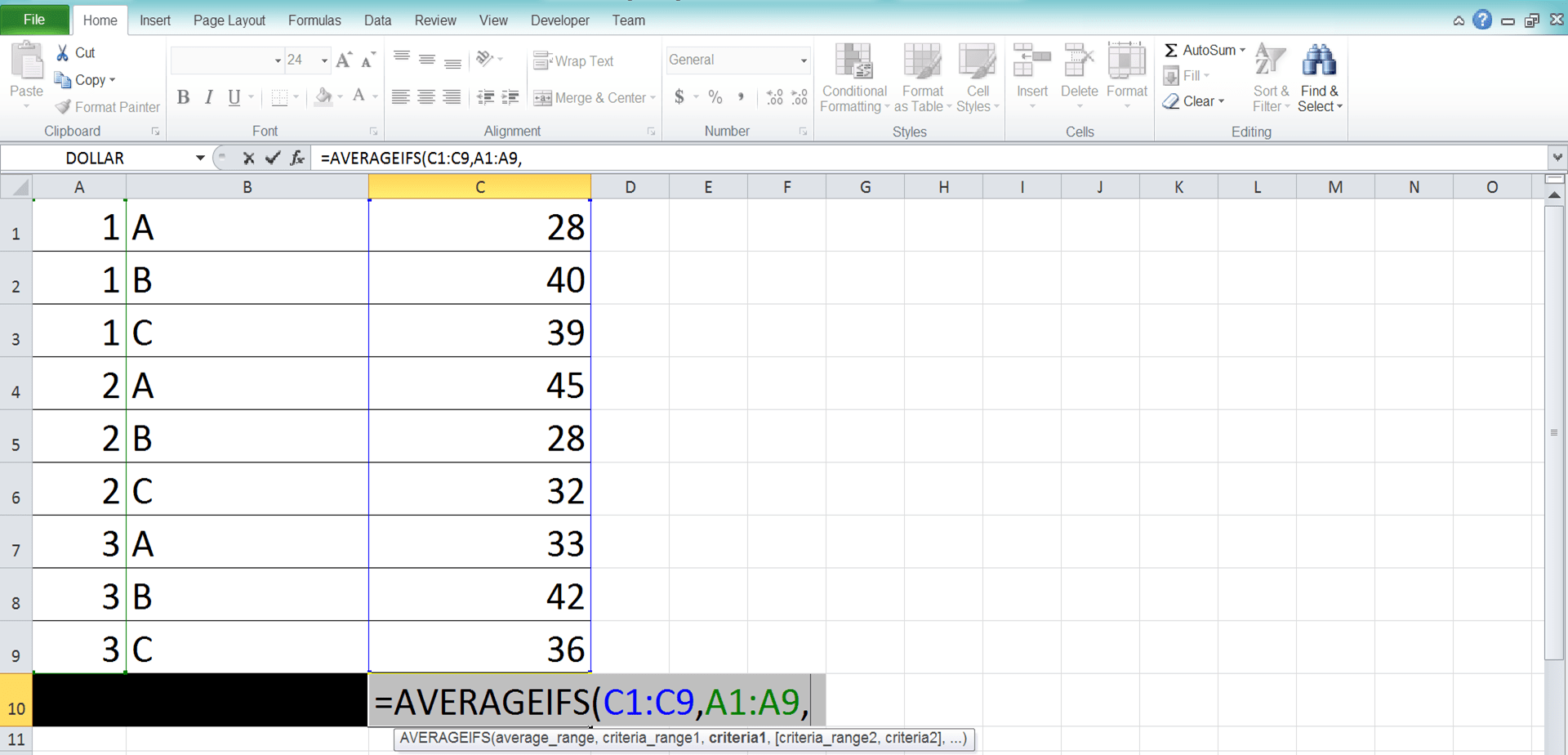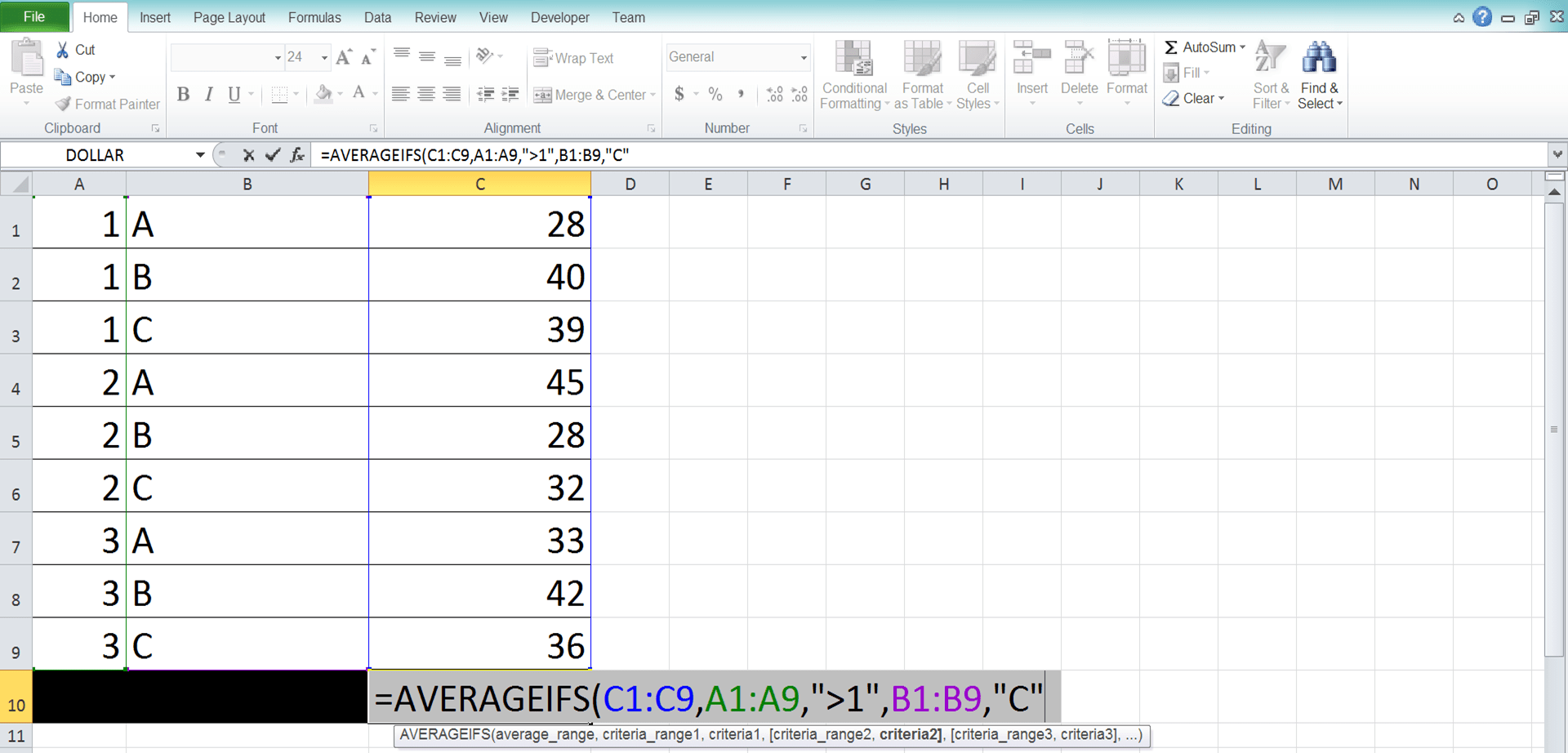How to Use the AVERAGEIFS Function in Excel: Usabilities, Examples, and Writing Steps - Compute Expert

# How to Use the AVERAGEIFS Function in Excel: Usabilities, Examples, and Writing Steps

Home >> Excel Tutorials from Compute Expert >> Excel Formulas List >> How to Use the AVERAGEIFS Function in Excel: Usabilities, Examples, and Writing Steps

In this tutorial, you will learn how to use the AVERAGEIFS function in excel completely.

When working with numbers in excel, we sometimes need to average numbers only from data entries that fulfill our criteria. We may have some number groups or classifications here that we must know the average of. If we know how to use AVERAGEIFS in excel, then we can get this kind of average we want fast.

Want to know more about AVERAGEIFS and how to use this formula properly in excel? Read this tutorial until its last part!

Disclaimer: This post may contain affiliate links from which we earn commission from qualifying purchases/actions at no additional cost for you. Learn more

## What is the AVERAGEIFS Function in Excel?

AVERAGEIFS function is an excel function that helps you to average numbers from data entries that fulfill your criteria.

## AVERAGEIFS Usability

You can use AVERAGEIFS to calculate the average of some selected numbers, instead of all numbers, in your cell range.

## AVERAGEIFS Result

AVERAGEIFS result is a number that represents the average of the numbers in which data entries fulfill your criteria.

## Excel Version from Which We Can Start Using AVERAGEIFS

We can start using AVERAGEIFS in excel since excel 2007.

## The Way to Write It and Its Inputs

Here is the general writing form of an AVERAGEIFS formula in excel.

= AVERAGEIFS ( number_range , data_range1 , criterion1 , … )

And here is a bit explanation of the inputs we need to give when we write an AVERAGEIFS
• number_range = the cell range (usually a column/row) where the numbers you want to average from your data entries are
• data_range1 = the cell range (usually a column/row) where the data you want to evaluate with your first criterion is. This cell range should be in parallel and has the same size as the number range
• criterion1 = the criterion that will evaluate each data in the cell range you input just before it
• = other pairs of data range and criteria that you have. You should input as many as the criteria you have for the data entries which number you want to average

AVERAGEIFS will only average the numbers in which data entry fulfills all the criteria you have.

## Criteria Writing in AVERAGEIFS

One important thing to pay attention to when you write your AVERAGEIFS in excel is the way you input your criteria. You should write them correctly in your AVERAGEIFS so you can get the correct average calculation result.

How to write the right criteria in AVERAGEIFS? You can find many examples of criteria writing in AVERAGEIFS together with their meaning below. Make sure to learn from them properly so you can write your AVERAGEIFS properly!

Text (not case-sensitive)
Criterion ExampleExplanation
"Jim"The same as “Jim”
“<>Jim”Not the same as “Jim”
“Jim*”With “Jim” prefix
“*jim”With “jim” suffix
“J*m”“J” prefix and “m” suffix
“Jim?”“Jim” prefix with any one character suffix
“?jim”Any one character prefix with “jim” suffix
“J?m”“J” prefix, any one character, and “m” suffix
“Jim~*”The same as “Jim*”
“Jim~?”The same as “Jim?”

A bit explanation of the symbols we use in the text criteria:
• * = any character with any amount
• ? = any one character
• ~ = used when you want to add * or ? character for the criterion

Number
Criterion ExampleExplanation
70Equal to 70
“>70”More than 70
“<70”Less than 70
“>=70”More than or equal to 70
“<=70”Less than or equal to 70

Date
Criterion ExampleExplanation
“>”&DATE(2019,12,3)Later than 3 December 2019

We don’t recommend inputting a date criterion with direct writing (e.g. “>3-12-2019”). Doing that can produce a wrong and unexpected result.

Cell coordinate
Criterion ExampleExplanation
“>”&B1More than the value in B1

Empty/non-empty cell
Criterion ExampleExplanation
“"Empty
“<>"Not empty

## Example of Its Usage and Result

Here is an implementation example of AVERAGEIFS in excel.In the example, we want to average the sales numbers of apples in region B from week 2 onwards. As we have criteria for the data entries numbers we want to average here, we use AVERAGEIFS.

As we discussed previously, we input the cell range where the numbers are first (sales quantity column) before inputting the data ranges and criteria. Remember that we need to input the data ranges and criteria in pairs in AVERAGEIFS. Thus, we input the week, product, and selling region columns side-by-side with their criterion. The data ranges here are in parallel and similar in size to the number range, just like what we need.

For the week, product, and selling region criteria, we input “>=2”, “Apple”, and “B”. This is similar to the pattern of the AVERAGEIFS criteria writing examples we have discussed before.

By writing the AVERAGEIFS correctly, we get the sales numbers average that we want, which is 707.6666667.

## Writing Steps

Confused when you need to write the AVERAGEIFS formula in your excel cell? See these AVERAGEIFS writing steps! We will discuss each step with an example screenshot to help you understand what to do in it easier!

1. Type an equal sign ( = ) in the cell where you want to put the AVERAGEIFS result2. Type AVERAGEIFS (can be with large and small letters) and an open bracket sign after =3. Input the cell range (usually a column/row) where the numbers you want to average from your data entries are. Then, type comma sign ( , )4. Input the cell range (usually a column/row) where the data you want to evaluate with your first criterion is. This cell range should be in parallel and has the same size as the numbers range we input before.

After we finish inputting the cell range, type a comma sign5. Input your first criterion that will evaluate each data in the cell range you just inputted6. Redo steps 4-5 until you have inputted all the data range and criterion pairs you need7. Type a close bracket sign8. Press Enter
9. Done!## AVERAGEIF vs AVERAGEIFS

You may have already averaged your numbers with AVERAGEIF before in excel. AVERAGEIF is also a formula you can use to average the numbers in your data entries that fulfill a criterion.

Then, what is the difference between AVERAGEIF and AVERAGEIFS? Well, the most important difference between the two is the number of criteria that AVERAGEIF and AVERAGEIFS can process. AVERAGEIF can only accept one criterion while AVERAGEIFS can accept more.

AVERAGEIFS looks to us like an upgrade version of AVERAGEIF although it isn’t (we can use both since excel 2007). You can also average with just one criterion with AVERAGEIFS. Thus, you may not need to use AVERAGEIF if you already have mastered AVERAGEIFS!

Another difference is the order of the inputs. In AVERAGEIF, you need to input the data range and criterion pair first before the number range. Meanwhile, you need to input the number range first in AVERAGEIFS.

In AVERAGEIF, the number range is also an optional input. If your number range is similar to your data range, then you only need to input the cell range once (in the data range input part). In AVERAGEIFS, however, you need to input both even though they are the same.

Choose the formula which suits your needs best when you need to average numbers from your data entries!

## Exercise

After you have understood how to use AVERAGEIFS in excel, now let’s sharpen your understanding through the following exercise!

### Questions

Use AVERAGEIFS to do the average calculation in each question:
1. What is the average of the sales quantities from A in region 1?
2. What is the average of the sales quantities from C with the indirect selling method?
3. What is the average of the sales quantities from A in region 2 with the direct selling method?

You can input up to 127 data range and criterion pairs in AVERAGEIFS.

Related tutorials you should learn:

Get updated excel info from Compute Expert by registering your email. It's free!